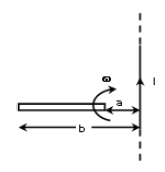# A copper rod moves with a constant angular velocity ω, about a long straight wire carrying a current i. If the ends of the rod from the wire are at distances a and b, then the e.m.f. induced in the rod isOption 1)Option 2)Option 3) zero Option 4)Motional EMF -- whereinmagnetic fieldlengthvelocity of u perpendicular to uniform magnetic field.

The velocity of any element and field applied by current carrying wire are parallel. Hence Emf induced is Zero.

Option 1)Option is incorrect

Option 2)Option is incorrect

Option 3)

zero

Option is correct

Option 4)Option is incorrect

Boost your Preparation for JEE Main 2021 with Personlized Coaching

Exams
Articles
Questions